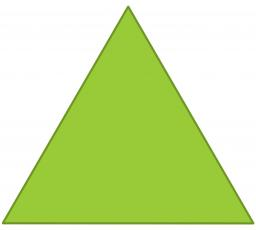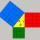# Height

Calculate height of the equilateral triangle if its perimeter is 8?

Correct result:

h =  2.3

#### Solution:We would be pleased if you find an error in the word problem, spelling mistakes, or inaccuracies and send it to us. Thank you!Tips to related online calculators
Pythagorean theorem is the base for the right triangle calculator.

#### You need to know the following knowledge to solve this word math problem:

We encourage you to watch this tutorial video on this math problem:

## Next similar math problems:

• Triangle eqCalculate accurate to hundredths cm height of an equilateral triangle with a side length 12 cm. Calculate also its perimeter and content area.
• Equilateral triangleCalculate the side of an equilateral triangle, if its area is 892 mm2.
• Square diagonalCalculate the length of the square diagonal if the perimeter is 476 cm.
• Height UTHow long is height in the equilateral triangle with a side b = 43?
• Hexagon areaThe center of the regular hexagon is 21 cm away from its side. Calculate the hexagon side and its area.
• Rhombus ABCDRhombus ABCD, |AC| = 90 cm, |BD| = 49 cm. Calculate the perimeter of the rhombus ABCD.
• Triangular pyramidA regular tetrahedron is a triangular pyramid whose base and walls are identical equilateral triangles. Calculate the height of this body if the edge length is a = 8 cm
• Right isoscelesCalculate area of the isosceles right triangle which perimeter is 41 cm.
• ArmCalculate the length of the arm r of isosceles triangle ABC, with base |AB| = 14 cm and a height v=18 cm.
• Similarity coefficientThe ratio of similarity of two equilateral triangles is 3.5 (ie 7:2). The length of the side of smaller triangle is 2.4 cm. Calculate the perimeter and area of ​​the larger triangle.
• Isosceles right triangleCalculate the area of an isosceles right triangle whose perimeter is 377 cm.
• Axial sectionAxial section of the cone is an equilateral triangle with area 168 cm2. Calculate the volume of the cone.
• OPTWhat is the perimeter of a right triangle with the legs 14 cm and 21 cm long?
• Height of pyramidThe pyramid ABCDV has edge lengths: AB = 4, AV = 7. What is its height?Ladder 8 m long is leaning against the wall. Its foot is 1 m away from the wall. In which height ladder touches the wall?Calculate the length of the diagonal AC of the rhombus ABCD, if its perimeter is 84 dm and the other diagonal BD has length 20 dm.Determine the volume of concrete block whose one edge of the base has a length 3 meters, body diagonal is 13 meters and its height is 12 meters.